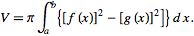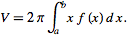# Area by washers and/or cylindrical shells: other than x,y

• tsamocki

#### tsamocki

What if you want to rotate around something other than the x/y axis?

For example:

## Homework Statement

y=x, y=0, x=1, rotated around the line x=-1

## Homework Equationsor## The Attempt at a Solution

V= ⌠(between 0 and 1)π[1+x]^2 dx

= π(1/3(x)^3+x^2+2x),x=0, x=1

=π((1/3)(1)^3+(1)^2+2(1))-0

= 10π/3

Shell method:

V= ⌠(between 0 and 1)2πx(x+1)dx

=2π((1/2)x^2+(1/3)x^3)x=1,x=0

=2π((1/2)+(1/3))-0

=2π(5/6)

=5π/3

Obviously the two answers do not match (i don't even know if i am correct on either); where am i going wrong?